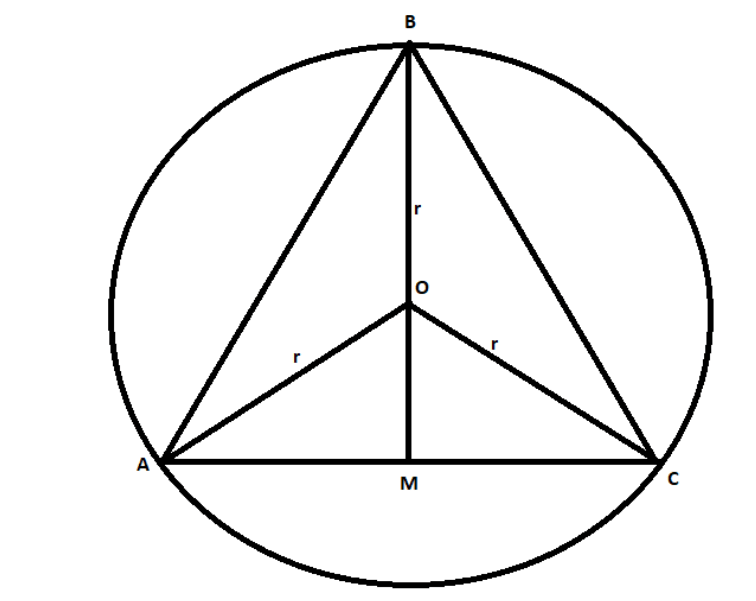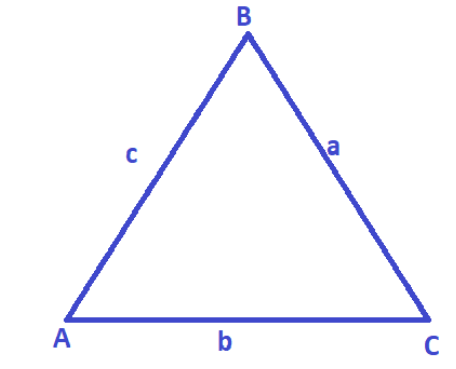Courses
Courses for Kids
Free study material
Offline Centres
MoreLast updated date: 01st Dec 2023
Total views: 281.1k
Views today: 3.81k

# How do you find the area of the equilateral triangle inscribed in a circle?Verified
281.1k+ views
Hint: We will consider a circle with a radius r and an equilateral triangle inscribed in it. We are going to use the sine rule to get the area of the triangle. Area of the triangle is the half of the product of base and height, so with the help of the rule we will obtain the value of base and height. Then the area of the triangle.

First let’s draw the given scenario,This is the overall situation. Now we know that $\vartriangle ABC$ is inscribed in the circle with radius r and center O. also that the given triangle is equilateral that has all angles measuring ${60^ \circ }$.
Now the formula to calculate the area of the triangle is,
$area\vartriangle = \dfrac{1}{2} \times base \times height$
Now in the triangle above we will take AC as the base and BM as the height of the triangle. But we need to find the dimensions of the base and height.
For that we will take the help of sine rule that relates the lengths of the sides of the triangle with the sines of the angles opposite to the side. That is in the above case;
$\dfrac{{AC}}{{\sin {{60}^ \circ }}} = \dfrac{{OA}}{{\sin {{30}^ \circ }}}$
So the value of AC is,
$AC = OA\dfrac{{\sin {{60}^ \circ }}}{{\sin {{30}^ \circ }}}$
Now we know that,
$\sin {60^ \circ } = \dfrac{{\sqrt 3 }}{2}\& \sin {30^ \circ } = \dfrac{1}{2}$
So substituting these values we get,
$AC = OA\dfrac{{\dfrac{{\sqrt 3 }}{2}}}{{\dfrac{1}{2}}}$
On calculating further,
$AC = OA\sqrt 3$
$AC = r\sqrt 3$ this is the value of base in the above case.
Now we need to find the value of BM.
We can observe from the figure above that, $BM = BO + OM$
Here BO is clearly visible as r but for OM;
In $\vartriangle OAM$,
$\sin {30^ \circ } = \dfrac{{{\text{Opposite}}}}{{{\text{Hypotenuse}}}} = \dfrac{{OM}}{r}$
So the value of OM is,
$OM = r.\sin {30^ \circ }$
Now putting both the values in the equation of BM,
$BM = r + r.\sin {30^ \circ }$
$BM = r + \dfrac{r}{2}$
Taking the LCM,
$BM = \dfrac{3}{2}r$
Now using the formula of area;
$A\left( {\vartriangle ABC} \right) = \dfrac{1}{2} \times \left( {\sqrt 3 r} \right) \times \dfrac{3}{2}r$
On calculating we get,
$A\left( {\vartriangle ABC} \right) = \dfrac{{3\sqrt 3 }}{4}{r^2}$
So, the correct answer is “$A\left( {\vartriangle ABC} \right) = \dfrac{{3\sqrt 3 }}{4}{r^2}$”.
If a, b and c are the three sides of the $\vartriangle ABC$then we can say that $\dfrac{a}{{\sin A}} = \dfrac{b}{{\sin B}} = \dfrac{c}{{\sin C}}$